# CHM2104 Lecture 2 (Hours 3-4).pptx

5 de Nov de 2022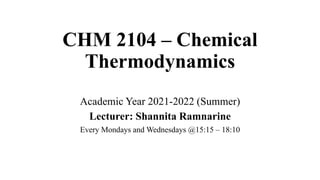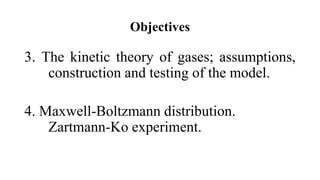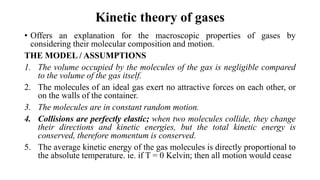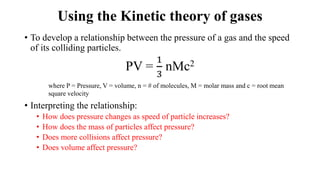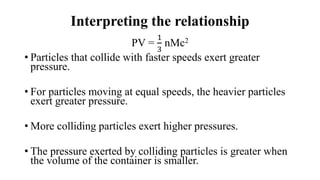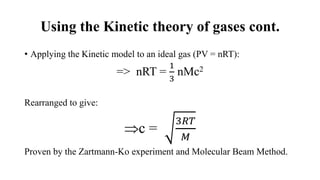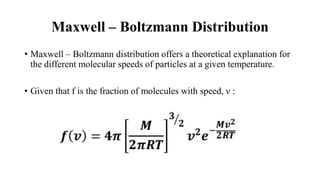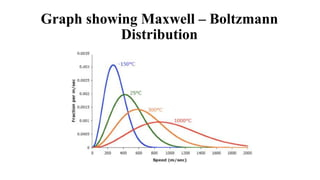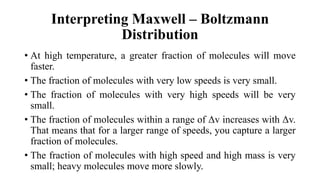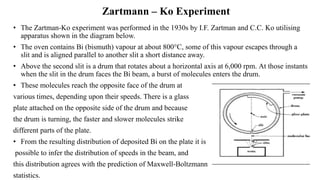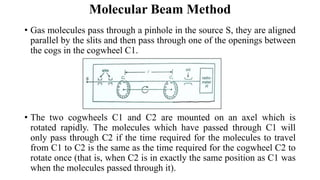1 de 11

### CHM2104 Lecture 2 (Hours 3-4).pptx

1. CHM 2104 – Chemical Thermodynamics Academic Year 2021-2022 (Summer) Lecturer: Shannita Ramnarine Every Mondays and Wednesdays @15:15 – 18:10
2. Objectives 3. The kinetic theory of gases; assumptions, construction and testing of the model. 4. Maxwell-Boltzmann distribution. Zartmann-Ko experiment.
3. Kinetic theory of gases • Offers an explanation for the macroscopic properties of gases by considering their molecular composition and motion. THE MODEL / ASSUMPTIONS 1. The volume occupied by the molecules of the gas is negligible compared to the volume of the gas itself. 2. The molecules of an ideal gas exert no attractive forces on each other, or on the walls of the container. 3. The molecules are in constant random motion. 4. Collisions are perfectly elastic; when two molecules collide, they change their directions and kinetic energies, but the total kinetic energy is conserved, therefore momentum is conserved. 5. The average kinetic energy of the gas molecules is directly proportional to the absolute temperature. ie. if T = 0 Kelvin; then all motion would cease
4. Using the Kinetic theory of gases • To develop a relationship between the pressure of a gas and the speed of its colliding particles. PV = 1 3 nMc2 where P = Pressure, V = volume, n = # of molecules, M = molar mass and c = root mean square velocity • Interpreting the relationship: • How does pressure changes as speed of particle increases? • How does the mass of particles affect pressure? • Does more collisions affect pressure? • Does volume affect pressure?
5. Interpreting the relationship PV = 1 3 nMc2 • Particles that collide with faster speeds exert greater pressure. • For particles moving at equal speeds, the heavier particles exert greater pressure. • More colliding particles exert higher pressures. • The pressure exerted by colliding particles is greater when the volume of the container is smaller.
6. Using the Kinetic theory of gases cont. • Applying the Kinetic model to an ideal gas (PV = nRT): => nRT = 1 3 nMc2 Rearranged to give: c = 3𝑅𝑇 𝑀 Proven by the Zartmann-Ko experiment and Molecular Beam Method.
7. Maxwell – Boltzmann Distribution • Maxwell – Boltzmann distribution offers a theoretical explanation for the different molecular speeds of particles at a given temperature. • Given that f is the fraction of molecules with speed, ν :
8. Graph showing Maxwell – Boltzmann Distribution
9. Interpreting Maxwell – Boltzmann Distribution • At high temperature, a greater fraction of molecules will move faster. • The fraction of molecules with very low speeds is very small. • The fraction of molecules with very high speeds will be very small. • The fraction of molecules within a range of Δv increases with Δv. That means that for a larger range of speeds, you capture a larger fraction of molecules. • The fraction of molecules with high speed and high mass is very small; heavy molecules move more slowly.
10. Zartmann – Ko Experiment • The Zartman-Ko experiment was performed in the 1930s by I.F. Zartman and C.C. Ko utilising apparatus shown in the diagram below. • The oven contains Bi (bismuth) vapour at about 800°C, some of this vapour escapes through a slit and is aligned parallel to another slit a short distance away. • Above the second slit is a drum that rotates about a horizontal axis at 6,000 rpm. At those instants when the slit in the drum faces the Bi beam, a burst of molecules enters the drum. • These molecules reach the opposite face of the drum at various times, depending upon their speeds. There is a glass plate attached on the opposite side of the drum and because the drum is turning, the faster and slower molecules strike different parts of the plate. • From the resulting distribution of deposited Bi on the plate it is possible to infer the distribution of speeds in the beam, and this distribution agrees with the prediction of Maxwell-Boltzmann statistics.
11. Molecular Beam Method • Gas molecules pass through a pinhole in the source S, they are aligned parallel by the slits and then pass through one of the openings between the cogs in the cogwheel C1. • The two cogwheels C1 and C2 are mounted on an axel which is rotated rapidly. The molecules which have passed through C1 will only pass through C2 if the time required for the molecules to travel from C1 to C2 is the same as the time required for the cogwheel C2 to rotate once (that is, when C2 is in exactly the same position as C1 was when the molecules passed through it).